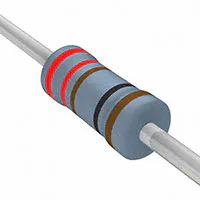Published

First, let's turn an LED on/off. Then, let's change the brightness of the LED!

BeginnerProtip30 minutes250## Things used in this project

### Hardware componentsArduino UNO & Genuino UNO
×1LED (generic)
×1×1Resistor 221 ohm
×1Jumper wires (generic)
×3

### Software apps and online servicesArduino IDE

## Code

### LED_Blinking - Change the brightness of LED (annoying ver.)

Arduino
The brightness of LED can change from 0 to 255. This version is more annoying than next cording.
```int led = 11;

void setup() {

pinMode(11, OUTPUT);

}

void loop() {

analogWrite(11, 0);
delay(1000);
analogWrite(11, 40);
delay(1000);
analogWrite(11, 80);
delay(1000);
analogWrite(11, 120);
delay(1000);
analogWrite(11, 160);
delay(1000);
analogWrite(11, 200);
delay(1000);
analogWrite(11, 250);
delay(1000);

}
```

### LED_Blinking - Change the brightness of LED (simple ver.)

Arduino
We have to use a functional formula. This method can be used when you do such a complicated job.

+ k++ means k=k+1. And it means k got 1 plus. So if you want to plus 2, insert k=k+2 instead of k++.
+ int k = random => random data from 0 to 255
```int led = 11;

void setup() {

pinMode(11, OUTPUT);

}

void loop() {
int k=0;
for (k=0; k<256; k++) {
analogWrite(11, k);
delay(10);
}

for (k=255; k>0; k--) {
analogWrite(11, k);
delay(10);
}

}
```

Arduino
You can change the time LED On or Off by data of delay.
1 second = 1000
```int led = 11;

void setup() {

pinMode(11, OUTPUT);

}

void loop() {

digitalWrite(11, HIGH);
delay(1000);
digitalWrite(11, LOW);
delay(1000);

}
```

### LED_Blinking - Change the brightness of LED (Serial something)

Arduino
If you open Arduino 1.8.5, you can find Serial Monitor. You can see some data device measured.
```int led = 11;

void setup() {
pinMode(led, OUTPUT);

Serial.begin(9600);

}

void loop() {
int i=0;
for(i=0; i<256; i++){
analogWrite(led, i);
Serial.print(i);
Serial.print("\n");
delay(10);
}

for(i=255; i>0; i--){
analogWrite(led, i);
Serial.print(i);
Serial.print("\n");
delay(10);
}
```

## Credits

### 채다은

1 project • 0 followers
Student, LYLM

### gledel

100 projects • 91 followers
Looking back on my childhood, I was happy when I was making something and I was proud of myself. "Making is instinct!"